# Solved Equations - Linear Equations Notes | Study Quantitative Reasoning for GMAT - GMAT

## GMAT: Solved Equations - Linear Equations Notes | Study Quantitative Reasoning for GMAT - GMAT

The document Solved Equations - Linear Equations Notes | Study Quantitative Reasoning for GMAT - GMAT is a part of the GMAT Course Quantitative Reasoning for GMAT.
All you need of GMAT at this link: GMAT

Section - 1
Ques 1: If x = 2, then x2 - 4x + 3 =
Ans:
x2 — 4x + 3 =
(2)2 - 4(2) + 3 =
4 - 8 + 3 = -1

Ques 2: If x = 3, what is the value of x +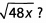Ans:
To evaluate the expression, replace x with (3).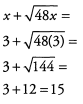Ques 3: What is the value of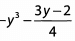when y= -2 ?
Ans:
To evaluate the expression, replace/with (-2) everywhere in the equation. Be extra careful with the negative signs.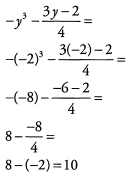Ques 4: If p = 300c2 - c, what is the value of p when c = 100?
Ans:
To find the value of p, we first need to replace c with 100.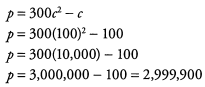Ques 5: What is the value of y if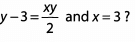Ans:
First we need to replace x with (3) in the equation.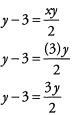Now, to find the value of y, we need to isolate y on one side of the equation.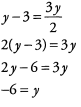Section - 2
Solve for the variable in the following equations.

Ques 6: 14 - 3x = 2
Ans: 14 -3x = 2
-3x = -12
x= 4
Subtract 14
Divide by -3

Ques 7: 3 (7 -x ) = 4(1.5)
Ans:
3(7 - x ) = 4(1.5)
21 - 3x = 6
- 3x = -15
x= 5
Simplify
Subtract 21
Divide by -3

Ques 8: .7x + 13 = 2x - 7
Ans:
7x + 13 = 2x — 7
5x+ 13 = -7
5x= -20
x = -4
Subtract 2x
Subtract 13
Divide by 5

Ques 9: 3t3 - 7 = 74
Ans:
3t3 - 7 = 74
3t3 = 81
t3 = 27
t= 3

Ques 10: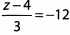Ans: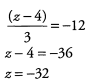Ques 11: 1,200x + 6,000 =13,200
Ans:
1,200x + 6,000= 13,200
1,200x= 7,200
x = 6

Ques 12: √x = 3 x 5 - 20 - 4
Ans: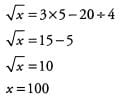Section - 3
Isolate x in the following equations.
Ques 13:
3x + 2(x + 2) = 2x + 16
Ans: 3x + 2(x+ 2) = 2x + 16
3x + 2x + 4 = 2x + 16
5x + 4 = 2x + 16
3x + 4 = 16
3x = 12
x = 4

Ques 14: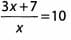Ans: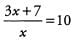3x + 7 = 10x
7 = 7x
1 = x

Ques 15: 4(-3x - 8) = 8(-x + 9)
Ans:
4 (-3x -8 ) = 8 (-x + 9)
-12 x - 32 = - 8x + 72
-32 = 4 x+ 72
-104 = 4x
-26 = x

Ques 16: 3x+ 7 - 4x + 8 = 2 ( - 2 x - 6)
Ans:
3x + 7 - 4x + 8 = 2 (-2x - 6)
-x + 15 = -4x - 12
3x + 15 = -12
3x = -27
x = -9

Ques 17: 2x(4 - 6) = -2 x + 12
Ans:
2x(4 - 6) = -2x + 12
2x(-2) = -2x + 12
-4x= -2x + 12
- 2x = 12
x = -6

Section - 4

Solve for the values of both variables in each system of equations using substitution. The explanations will use substitution to solve.
Ques 18: 7x - 3y = 5
y= 10
Ans:
7x - 3y = 5,y= 10
7x - 3(10) = 5
7x - 30 = 5
7x = 35
x = 5

Ques 19: y = 4x+ 10
y = 7 x - 5
Ans:
(4x+10) = 7x - 5
10 = 3x - 5
15 = 3x
5 = x
7 = 4(5) + 10
y - 30
Answer:x = 5, y = 30

Ques 20: 2h-4k = 0,k = h - 3
Ans:
2 h - 4 ( h - 3 ) = 0
2h - 4h +12 =0
-2h = - 12
h = 6
k= (6) - 3
k = 3
Answer: h = 6, k = 3

Section - 5
Solve for the values of both variables in each system of equations using elimination. The explanations will use elimination to solve.
Ques 21: x -y = 4

2 x + y = 5
Ans:
Notice that the first equation has the term -y while the second equation has the term +7 . Because these terms will cancel, we do not need to do any manipulations before adding the equations together: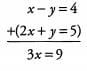Therefore x = 3 and plugging this back in to the first equation yields: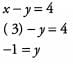Answer: x - 3 and 7 = —1

Ques 22: x + 2y =5
x-4y = -7
Ans:
Both equations have the term +x, so we can eliminate the variable x by subtracting the second equation from the first: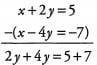The new equation simplifies to 67 = 12, or7 = 2. Then, we plug this value for7 into the first equation to get x + 2(2) = 5, or x = 1.
Notice that we must be very careful to change the sign of each term in the second equation when subtracting. Alternatively, we could have multiplied the entire second equation by -1 to get -x+4 y = 7 and then added this equation to the first: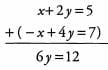This yields the same solution: y = 2 and x = 1.

Ques 23: a + d = 8,2a + b = 13
Ans:
Both equations have the term +b, so we can eliminate the variable b by subtracting the second equation from the first: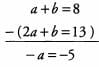Hence a = 5. Then, we plug this value for a into the first equation to get (5) + b = 8, or b = 3.
Answer: a = 5, b = 3

Section - 6
Solve for the values of both variables in each system of equations. Decide whether to use substitution or elimination. Explanation will use one of the two methods and explain why that is the better solution method.
Ques 24:
5 x + 2 y = 12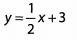Ans: When one of the two equations is already solved for one of the variables, substitution is almost always the better method. In this particular problem, the second equation is solved for y, so we take the right-hand side of this equation and substitute it for y in the first equation: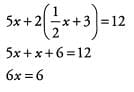Therefore x = 1. We can now plug this value for x in to either of the original equations to solve for y, but it will be easiest to plug in to the equation that was used for the substitution (after all, it is already solved for y). Hence y = (1/2) x (1) + 3 = 3.5.
Answer: x = 1, y = 3.5

Ques 25: y -1 =x + 2
2y = x + 1

Ans: For this system of equations, either method would be appropriate. Both equations would require some manipulation before we could simply stack-and-add and neither equation is already solved for one of its variables. When neither method seems to have an advantage, pick whichever you like best.
If we use substitution, it is best to solve the first equation for y, giving us y = x + 3, and then substitute this into the second equation: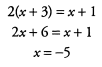We then plug this into the equation used for the substitution step to get y = (-5) + 3 = -2.
Answer: x = -5 , y = -2

The document Solved Equations - Linear Equations Notes | Study Quantitative Reasoning for GMAT - GMAT is a part of the GMAT Course Quantitative Reasoning for GMAT.
All you need of GMAT at this link: GMATUse Code STAYHOME200 and get INR 200 additional OFF

## Quantitative Reasoning for GMAT

75 videos|87 docs|156 tests

Track your progress, build streaks, highlight & save important lessons and more!

,

,

,

,

,

,

,

,

,

,

,

,

,

,

,

,

,

,

,

,

,

;